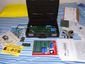# Apple II SCIENTIFIC CALCULATOR by MATTEO TREVISAN

1 post / 0 newOffline
Last seen: 7 months 3 days ago
Joined: Nov 21 2010 - 15:30
Posts: 248
Apple II SCIENTIFIC CALCULATOR by MATTEO TREVISAN

Hello, This is an implementation of my Apple II SIMPLY CALCULATOR to be a SCIENTIFIC CALCULATOR ONE, Here the code:

5 SPEED = 150
6 INVERSE
10 PRINT "APPLE ][ SCIENTIFIC CALCULATOR 1.0 BY MATTEO TREVISAN"
20 HOME
30 PRINT "DO YOU WANT TO ADD,SUBTRACT,DIVIDE, MULTIPLY, SQUARE ROOT, EXPONENTIATION , PERCENTAGE, TANGENT, LOGARITHM, SINE OR COSINE":INPUT A\$
40 IF A\$ = "ADD" THEN GOTO 120
50 IF A\$ = "SUBTRACT" THEN GOTO 170
60 IF A\$ = "DIVIDE" THEN GOTO 220
70 IF A\$ = "MULTIPLY" THEN GOTO 270
80 IF A\$ = "SQUARE ROOT" THEN GOTO 330
81 IF A\$ = "EXPONENTIATION" THEN GOTO 390
82 IF A\$ = "PERCENTAGE" THEN GOTO 470
83 IF A\$ = "PUDDING" THEN PRINT "DO YOU WANT SOME PUDDING?"
84 IF A\$ = "SINE" THEN GOTO 550
85 IF A\$ = "COSINE" THEN GOTO 620
86 IF A\$ = "TANGENT" THEN GOTO 690
87 IF A\$ = "LOGARITHM" THEN GOTO 760
90 IF A\$ <> "ADD" THEN GOTO 10
91 IF A\$ <> "SUBTRACT" THEN GOTO 10
92 IF A\$ <> "DIVIDE" THEN GOTO 10
93 IF A\$ <> "MULTIPLY" THEN GOTO 10
94 IF A\$ <> "SQUARE ROOT" THEN GOTO 10
95 IF A\$ <> "EXPONENTIATION" THEN GOTO 10
96 IF A\$ <> "PERCENTAGE" THEN GOTO 10
97 IF A\$ <> "SINE" THEN GOTO 10
98 IF A\$ <> "COSINE" THEN GOTO 10
99 IF A\$ <> "TANGENT" THEN GOTO 10
100 IF A\$ <> "LOGARITHM" THEN GOTO 10
120 PRINT "TELL ME THE NUMBER TO ADD"
130 PRINT "NUMBER ONE":INPUT B
140 PRINT "NUMBER TWO":INPUT C
150 D = B+C
160 PRINT "THE ADDITION IS "; D
161 FOR K = 1 TO 5000
162 NEXT K
165 GOTO 10
170 PRINT "TELL ME THE NUMBER TO SUBTRACT"
180 PRINT "NUMBER ONE":INPUT E
190 PRINT "NUMBER TWO":INPUT F
200 G = E-F
210 PRINT "THE SUBTRACTION IS "; G
211 FOR K = 1 TO 5000
212 NEXT K
215 GOTO 10
220 PRINT "TELL ME THE NUMBER TO DIVIDE"
230 PRINT "NUMBER ONE":INPUT H
240 PRINT "NUMBER TWO":INPUT I
241 IF I = 0 THEN GOTO 265
250 L = H/I
260 PRINT "THE DIVISION IS "; L
262 FOR K = 1 TO 5000
263 NEXT K
265 GOTO 10
270 PRINT "TELL ME THE NUMBER TO MULTIPLY"
280 PRINT "NUMBER ONE":INPUT M
290 PRINT "NUMBER TWO":INPUT N
300 O = M*N
310 PRINT "THE MULTIPLY IS "; O
311 FOR K = 1 TO 5000
312 NEXT K
315 GOTO 10
320 REM THIS PART OF THE PROGRAM WAS ADDED BY INSANITOR
330 PRINT "TELL ME THE NUMBER TO SQUARE ROOT"
335 PRINT "NUMBER":INPUT Q
350 PRINT "THE SQUARE ROOT IS ";SQR(Q)
360 FOR K = 1 TO 5000
370 NEXT K
380 GOTO 10
390 PRINT "TELL ME THE NUMBER YOU WANT THE EXPONENTIATION"
400 PRINT "NUMBER ONE":INPUT P
410 PRINT "NUMBER TWO":INPUT Q
420 R = P^Q
430 PRINT "THE EXPONENTIATION IS "; R
440 FOR K = 1 TO 5000
450 NEXT K
460 GOTO 10
470 PRINT "TELL ME THE PERCENTAGE TO CALCULATE"
480 PRINT "NUMBER ONE %":INPUT S
490 PRINT "NUMBER TWO":INPUT T
500 U = (S/100)*T
510 PRINT "THE PERCENTAGE IS "; U
520 FOR K = 1 TO 5000
530 NEXT K
540 GOTO 10
550 PRINT "TELL ME THE NUMBER TO SINE"
560 PRINT "INSERT NUMBER":INPUT V
570 Z = SIN(V)
580 PRINT "SINE IS "; Z
590 FOR K = 1 TO 5000
600 NEXT K
610 GOTO 10
620 PRINT "TELL ME THE NUMBER TO COSINE"
630 PRINT "INSERT NUMBER":INPUT Y
640 W = COS(Y)
650 PRINT "COSINE IS "; W
660 FOR K = 1 TO 5000
670 NEXT K
680 GOTO 10
690 PRINT "TELL ME THE NUMBER TO TANGENT"
700 PRINT "INSERT NUMBER":INPUT SA
710 SB = TAN(SA)
720 PRINT "TAN IS "; SB
730 FOR K = 1 TO 5000
740 NEXT K
750 GOTO 10
760 PRINT "TELL ME THE NUMBER TO LOGARITHM"
770 PRINT "INSERT NUMBER":INPUT SC
780 SD = LOG(SC)
790 PRINT "LOGARITHM IS "; SD
800 FOR K = 1 TO 5000
810 NEXT K
820 GOTO 10
830 END

And here you can download a .dsk disk image file for your Apple II emulator or real machine.